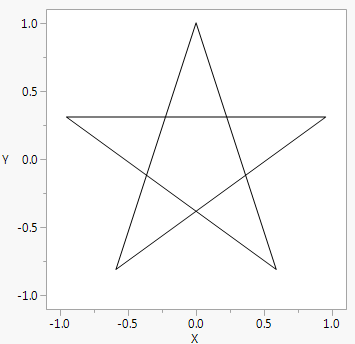Publication date: 11/10/2021

## Draw Lines

Line() draws lines between points.

`win = New Window( "Five-Point Star",`
`	Graph Box(`
`		Frame Size( 300, 300 ),`
`		X Scale( -1.1, 1.1 ),`
`		Y Scale( -1.1, 1.1 ),`
`		Line(`
`			{Cos( 1 * Pi() / 10 ), Sin( 1 * Pi() / 10 )},`
`			{Cos( 9 * Pi() / 10 ), Sin( 9 * Pi() / 10 )},`
`			{Cos( 17 * Pi() / 10 ), Sin( 17 * Pi() / 10 )},`
`			{Cos( 5 * Pi() / 10 ), Sin( 5 * Pi() / 10 )},`
`			{Cos( 13 * Pi() / 10 ), Sin( 13 * Pi() / 10 )},`
`			{Cos( 1 * Pi() / 10 ), Sin( 1 * Pi() / 10 )}`
`		)`
`	)`
`);`

Figure 12.10 Using Lines to Draw a StarYou can either specify the points in two-item lists as demonstrated above or as matrices of x and then y coordinates. Matrices are flattened by rows. You can use either row or column vectors, as long as you have the same number of elements in each matrix. The following expressions have the same effect:

`Line( {1,2}, {3,0}, {2,4} ); // several {x,y} lists`
`Line( [1 3 2],[2 0 4] ); // row vectors`
`Line( [1,3,2], [2,0,4] ); // column vectors`
`Line( [1 3 2], [2,0,4] ); // one of each`

The star example could also be drawn this way. Note that the script must include the full Matrix( {...} ) notation rather than [ ] shorthand because the entries are expressions. The following example uses the Matrix() function.

`win = New Window( "Five-Point Star",`
`	Graph Box(`
`		Frame Size( 300, 300 ),`
`		X Scale( -1.1, 1.1 ),`
`		Y Scale( -1.1, 1.1 ),`
`		Line(`
`			Matrix(`
`				{ // the x coordinates`
`				Cos( 1 * Pi() / 10 ), Cos( 9 * Pi() / 10 ), Cos( 17 * Pi() / 10 ),`
`				Cos( 5 * Pi() / 10 ), Cos( 13 * Pi() / 10 ), Cos( 1 * Pi() / 10 )}`
`			),`
`			Matrix(`
`				{ // the y coordinates`
`				Sin( 1 * Pi() / 10 ), Sin( 9 * Pi() / 10 ), Sin( 17 * Pi() / 10 ),`
`				Sin( 5 * Pi() / 10 ), Sin( 13 * Pi() / 10 ), Sin( 1 * Pi() / 10 )}`
`			)`
`		) ) );`

HLine() draws a horizontal line across the graph at the y-value that you specify. Similarly, VLine() draws a vertical line down the graph at the x-value that you specify. Both functions support drawing multiple lines by using a matrix of values in the y argument. See Mousetrap().